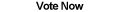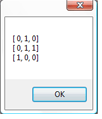Home Articles Components Code Snippets Links# C# Boolean Matrix## Boolean Matrix

A Boolean Matrix in C# (also known as a logical matrix) is a useful mathematical tool for complex math operations. A boolean matrix is a number matrix that consists of only 1's and 0's (or true and false). They are useful in a wide range of mathematics.

Since .NET Framework does not come with built-in support for matrix math, our boolean matrix C# math library is going to be written from scratch.

## Representing the Matrix

The C# matrix will be represented using a multi-dimensional array. Multi-dimensional arrays in C# are denoted by a comma between brackets, such as [,]. There are advantages and disadvantages of using multi-dimensional arrays vs jagged arrays ([][] for example). In our case, multi-dimensional arrays make the C# programming a bit simpler.

## Matrix Operations

Our C# Boolean Matrix supports three operations: Meet, Join, and Product. Meet and Join are the simplest matrix operations since they only work between matrices of the same dimensions. Meet "and's" each element between the matrices while Join "or's" them.

Product is a bit more complicated. The product operation is regular matrix multiplication. In a nutshell, only boolean matrices where the number of columns in one is the same as the number of rows in the other can be multiplied.

## Matrix Closures

Closures in a boolean matrix as used if the matrix represents a relation. This is something pretty mathy, but C# makes it very simple to program. The closure algorithms included are Reflexive, Symmetric, and Transitive. Transitive closure is the most complex and it uses Warshall's Algorithm to calculate the transitive closure of any boolean matrix.

## Matrix Transformations

The only matrix transformation included in the C# class is Transpose. Matrix transponse basically flips the width and height of a matrix, making the rows into columns (or columns into rows).

My intention was to include an Inverse transformation C# function, but the problem is too complex for our simple boolean matrix code.

## The Source Code

The C# source code for the boolean matrix class is straight foward. As mentioned before, I opted for a multi-dimensional array to store the values to make the code more readable. In that note, the matrix class also includes a simple algorithm to make the matrix readable on-screen (called with ToString).

Back to C# Article List